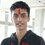# A Recursive Sum

I was playing around with recursions and I found something interesting which I would like to share with you guys. Do give it a try.

We recursively define $a_n=\dfrac{a_{n-2}}{n}$. Also, $a_0=a_1=1$. Prove that $\displaystyle\sum_{n=0}^{\infty}a_n=\sqrt{\dfrac{e\pi}{2}}\mathrm{erf}\left(\dfrac{1}{\sqrt{2}}\right)+\sqrt{e}$Note by Pratik Shastri
6 years, 4 months ago

This discussion board is a place to discuss our Daily Challenges and the math and science related to those challenges. Explanations are more than just a solution — they should explain the steps and thinking strategies that you used to obtain the solution. Comments should further the discussion of math and science.

When posting on Brilliant:

• Use the emojis to react to an explanation, whether you're congratulating a job well done , or just really confused .
• Ask specific questions about the challenge or the steps in somebody's explanation. Well-posed questions can add a lot to the discussion, but posting "I don't understand!" doesn't help anyone.
• Try to contribute something new to the discussion, whether it is an extension, generalization or other idea related to the challenge.

MarkdownAppears as
*italics* or _italics_ italics
**bold** or __bold__ bold
- bulleted- list
• bulleted
• list
1. numbered2. list
1. numbered
2. list
Note: you must add a full line of space before and after lists for them to show up correctly
paragraph 1paragraph 2

paragraph 1

paragraph 2

[example link](https://brilliant.org)example link
> This is a quote
This is a quote
    # I indented these lines
# 4 spaces, and now they show
# up as a code block.

print "hello world"
# I indented these lines
# 4 spaces, and now they show
# up as a code block.

print "hello world"
MathAppears as
Remember to wrap math in $$ ... $$ or $ ... $ to ensure proper formatting.
2 \times 3 $2 \times 3$
2^{34} $2^{34}$
a_{i-1} $a_{i-1}$
\frac{2}{3} $\frac{2}{3}$
\sqrt{2} $\sqrt{2}$
\sum_{i=1}^3 $\sum_{i=1}^3$
\sin \theta $\sin \theta$
\boxed{123} $\boxed{123}$

Sort by:

My method is the method of generating functions :

$\displaystyle y=f(x)=\sum _{ n=0 }^{ \infty }{ { a }_{ n }{ x }^{ n } }$

Remember our recurrence relation is :

$\displaystyle (n+2){ a }_{ n+2 }={ a }_{ n }$

Also $f(x)$ can be written as :

$\displaystyle f(x) = { a }_{ 0 }+{ a }_{ 1 }x+\sum _{ n=2 }^{ \infty }{ { a }_{ n }{ x }^{ n } }$

Since ${a}_{0} = {a}_{1} =1$

$\displaystyle \Rightarrow f(x) = 1+x+\sum _{ n=0 }^{ \infty }{ { a }_{ n+2 }{ x }^{ n+2 } }$

Differentiating both sides with respect to $x$ we have :

$\displaystyle f'(x)=1+\sum _{ n=0 }^{ \infty }{ (n+2){ a }_{ n+2 }{ x }^{ n+1 } }$

Using our recurrence relation we have :

$\displaystyle f'(x)=1+\sum _{ n=0 }^{ \infty }{ { a }_{ n }{ x }^{ n+1 } }$

$\displaystyle \Rightarrow f'(x)=1+xf(x)$

Now it's a linear differential equation and it's solution is as follows :

$\displaystyle y{ e }^{ -{ x }^{ 2 }/2 }=\int _{ 0 }^{ x }{ { e }^{ -{ t }^{ 2 }/2 }dt } + C$

Now at $x=0 , y=1$ hence it comes out to be $C=1$

Finally $\displaystyle y = f(x) ={ e }^{ { x }^{ 2 }/2 }\int _{ 0 }^{ x }{ { e }^{ -{ t }^{ 2 }/2 }dt } +{ e }^{ { x }^{ 2 }/2 }$

To get the value of summation simply put $x=1$ to get :

$\displaystyle \sum _{ n=0 }^{ \infty }{ { a }_{ n } } =\sqrt { e } \int _{ 0 }^{ 1 }{ { e }^{ -{ t }^{ 2 }/2 }dt } +\sqrt { e }$

With a little change of variables we have :

$\displaystyle \sum _{ n=0 }^{ \infty }{ { a }_{ n } } =\sqrt { 2e } \int _{ 0 }^{ 1/\sqrt { 2 } }{ { e }^{ -{ x }^{ 2 } }dx } +\sqrt { e }$

Using the defintion of error function we have finally :

$\displaystyle \int _{ 0 }^{z}{ { e }^{ -{ x }^{ 2 } }dx } = \frac { \sqrt { \pi } }{ 2 } \mathrm{erf}(z)$

$\displaystyle \sum _{ n=0 }^{ \infty }{ { a }_{ n } } =\sqrt { \frac { e\pi }{ 2 } } \mathrm{erf}(\frac { 1 }{ \sqrt { 2 } } )+\sqrt { e }$

- 6 years, 4 months ago

Excellent solution as usual. And now thanks to You I learned how to solve infinite sums using generating functions.

- 6 years, 4 months ago

Wonder if there is another way to get to the sum..

- 6 years, 4 months ago

There is an another way you can solve for the general term and then sum can be calculated very easily.

- 6 years, 4 months ago

The general term looks horrid. How do we sum it?

- 6 years, 4 months ago

Nice ! +1

- 6 years, 4 months ago

Cool! That's exactly what I did too! Ditto! +1!

- 6 years, 4 months ago